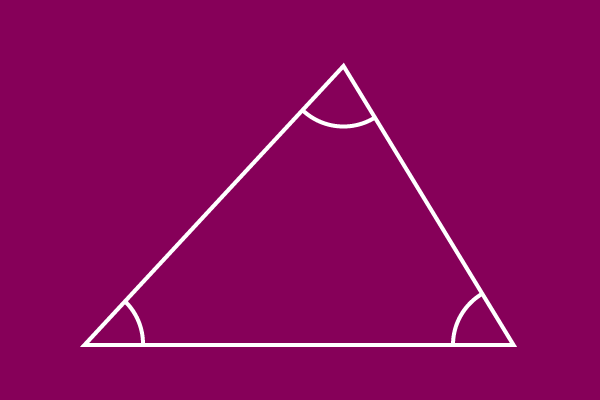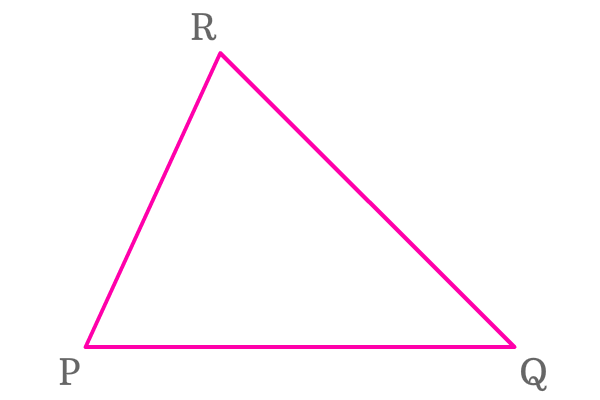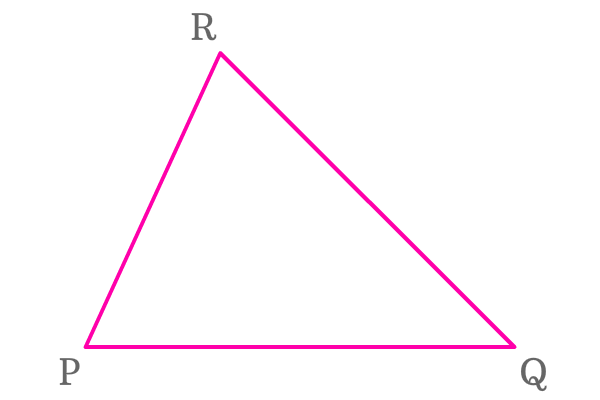# Interior Angles of a Triangle## Definition

An angle, which formed inside the triangle at a point by the meeting of any two of three sides is called an interior angle of the triangle.

Three sides form three angles inside the triangle by the meeting of every two sides. The three angles are actually formed inside the triangle. Hence, they are called as interior angles of the triangle.

### List of interior angles

$\Delta PQR$ is a triangle and its sides are $\overline{PQ}$, $\overline{QR}$ and $\overline{RP}$. These three sides form three interior angles in the triangle.

1
###### First Interior angleThe sides $\overline{PR}$ and $\overline{PQ}$ formed an angle inside the triangle at corresponding vertex $P$.

The angle ($\angle RPQ$) is called an interior angle of the triangle $PQR$.

This interior angle is denoted in three forms.

$\angle RPQ = \angle QPR = \angle P$

2
###### Second Interior angleThe sides $\overline{PQ}$ and $\overline{QR}$ formed an angle inside the triangle at respective vertex $Q$.

The angle ($\angle PQR$) is called as an interior angle of the triangle $PQR$.

This interior angle can be expressed in three different forms.

$\angle PQR = \angle RQP = \angle Q$

3
###### Third Interior angleThe sides $\overline{PR}$ and $\overline{QR}$ formed an angle inside the triangle at associated vertex $R$.

The angle ($\angle PRQ$) is called an interior angle of the $\Delta PQR$.

This interior angle can be represented in three various forms.

$\angle PRQ = \angle QRP = \angle R$

Latest Math Topics
Latest Math Problems
Email subscription
Math Doubts is a best place to learn mathematics and from basics to advanced scientific level for students, teachers and researchers. Know more
Follow us on Social Media
###### Math Problems

Learn how to solve easy to difficult mathematics problems of all topics in various methods with step by step process and also maths questions for practising.